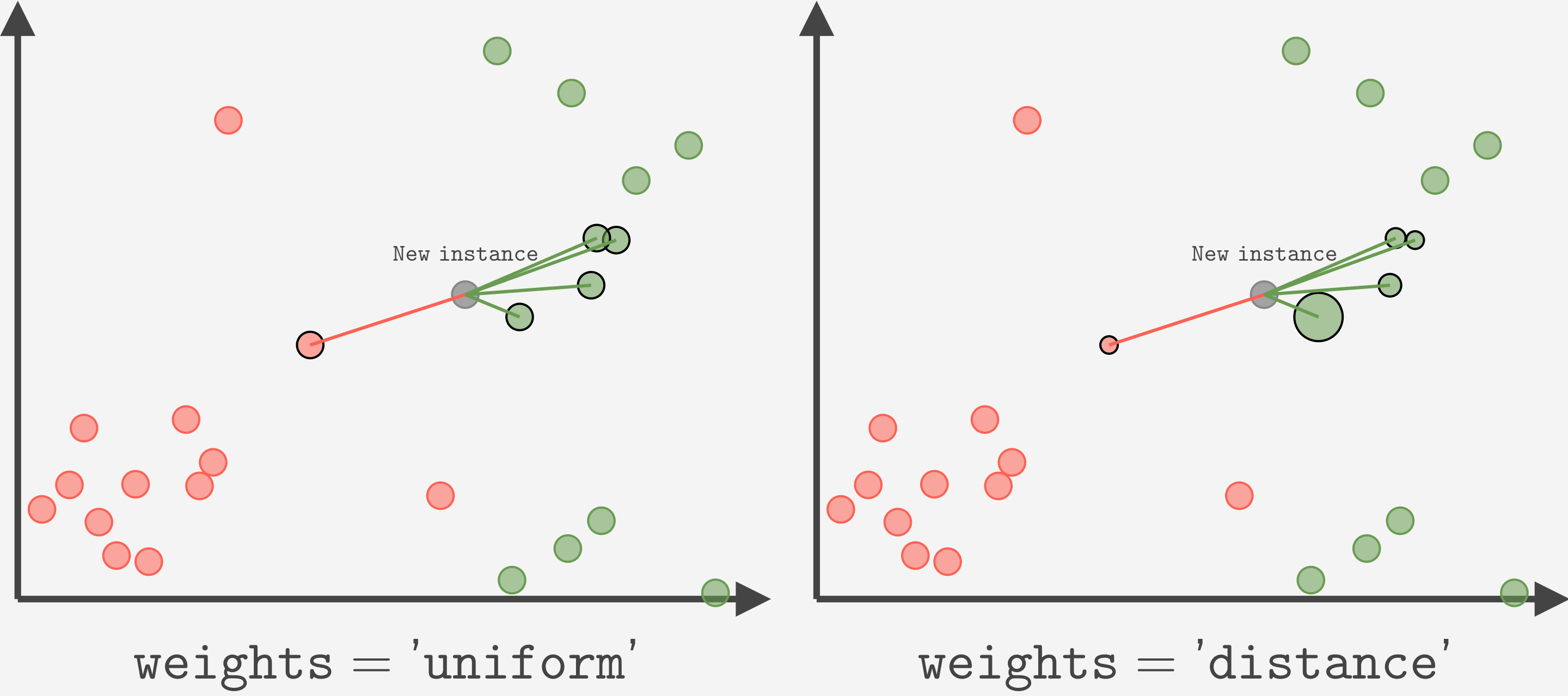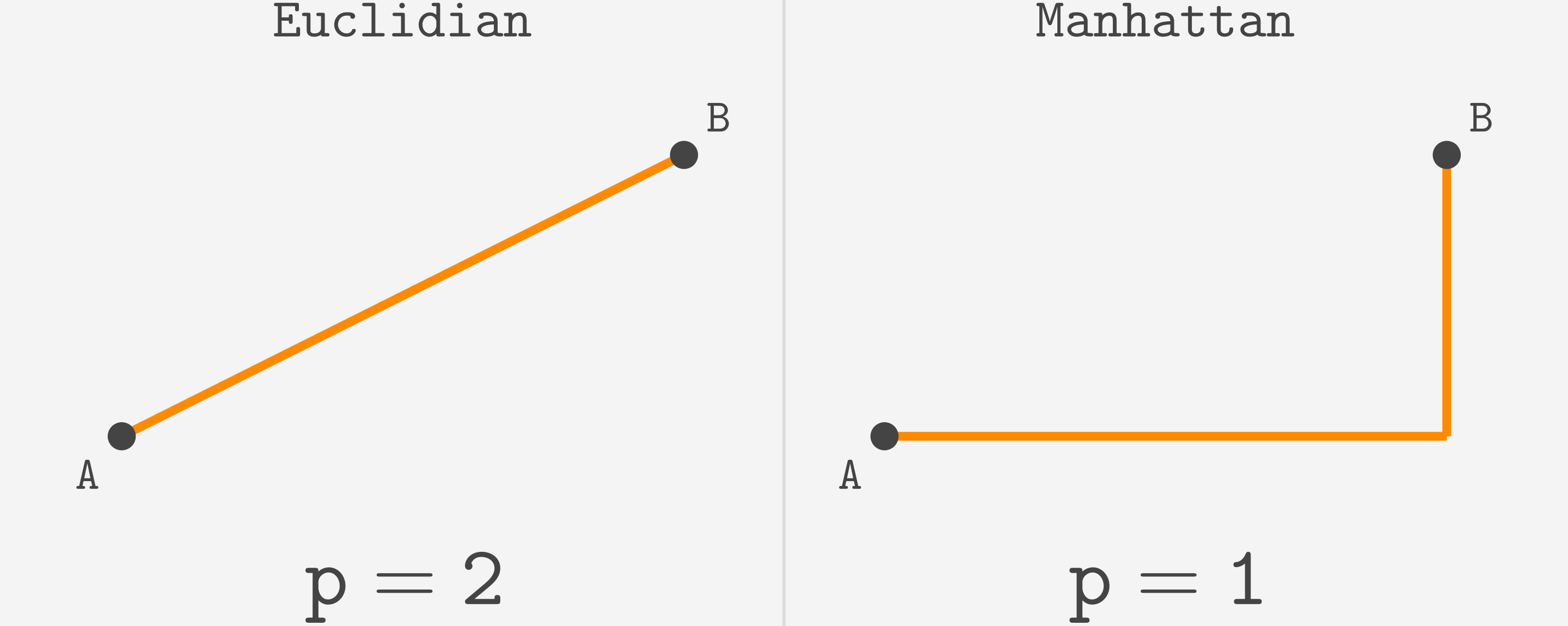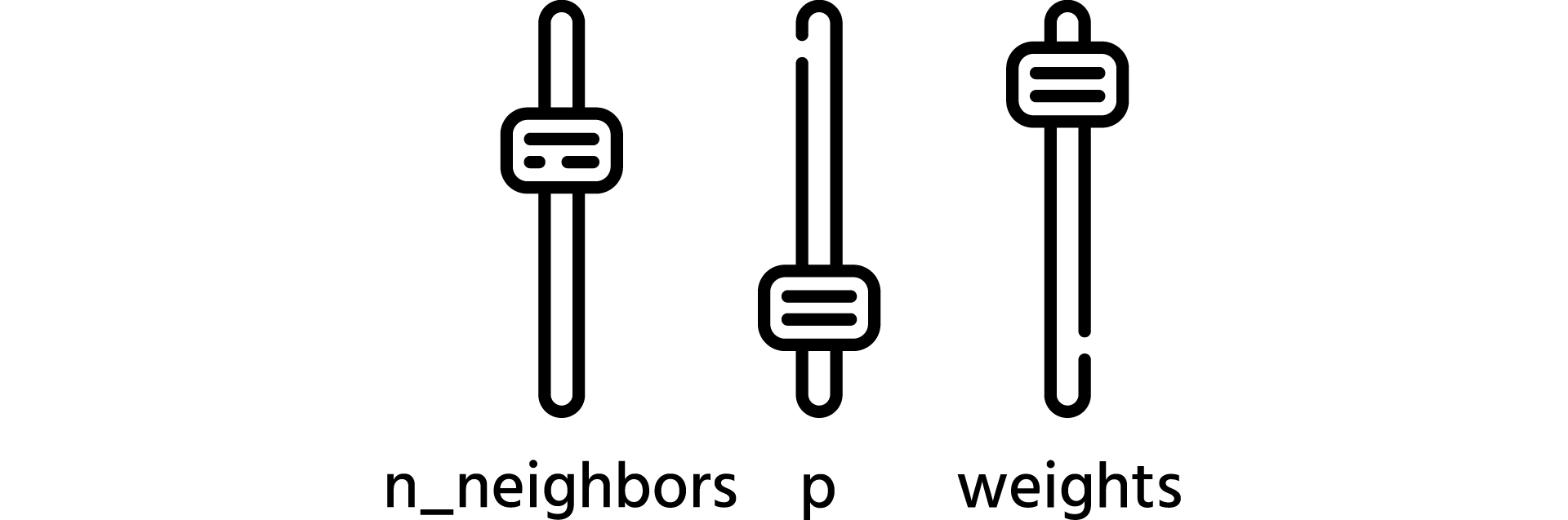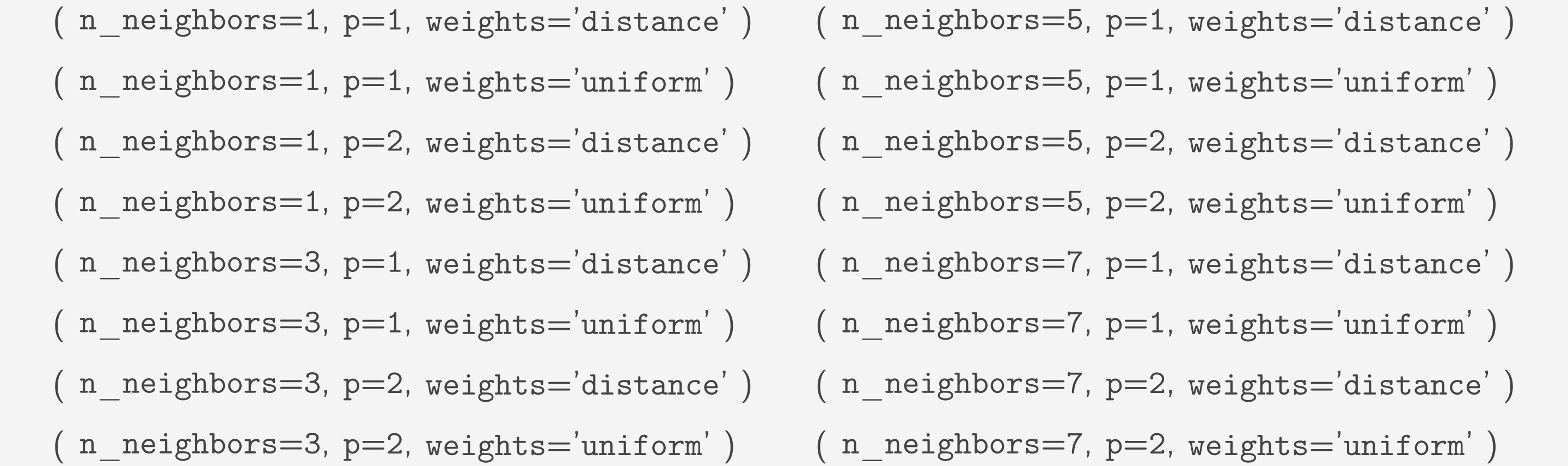Course Content

ML Introduction with scikit-learn

##The Flaw of GridSearchCV

Before we talk about `GridSearchCV`, it needs to be mentioned that `KNeighborsClassifier` has more than 1 hyperparameter to tweak.
By now, we only used `n_neighbors`. Let's shortly discuss two other hyperparameters, `weights` and `p`.

#### weights

As you probably remember, `KNeighborsClassifier` works by finding the k nearest neighbors.
Then it assigns the most frequent class among those neighbors irrespective of how close each one is.
Another approach is to also consider the distance to that neighbor so that the closer neighbors' classes have more weight.
This can be done by setting the `weights='distance'`.
By default, the first approach is used, which is set using `weights='uniform'`.#### p

There are also different ways to calculate the distance. `p` hyperparameter controls it.
Let's illustrate how the distance is calculated for `p=1` and `p=2`.
`p=1` is a Manhattan distance.
`p=2` is a Euclidian distance that you learned in school.A `p` parameter can take any positive integer. There are many different distances, but they are harder to visualize than `p=1` or `p=2`.

Note

Don't worry if you did not understand what `weights` or `p` is for or how it works.
They are briefly explained just to show that there is more than one hyperparameter that may change the model's predictions.
You can think of them as just some hyperparameters we can tune.In the last chapter, we used `GridSeachCV` to find the best value of `n_neighbors`.
What if we wanted to find the best combination of `n_neighbors`, `weights`, and `p`? Well, the `param_grid` would look like this:

`GridSearchCV` tries all the possible combinations to find the best.
So it will try all of those:That's already a lot of work. But what if we want to try more values? For example,

Now there are 100 combinations. And remember that we need to train and evaluate a model 5 times to get its cross-validation score.
So this process is done 500 times.
It is not a problem for our tiny dataset, but usually, datasets are much larger, and training may take a lot of time.
Doing this process 500 times is painfully slow in that case. That's why `RandomizedSearchCV` is used more often for larger datasets.
The next chapter will explain what it is and give you some practice!